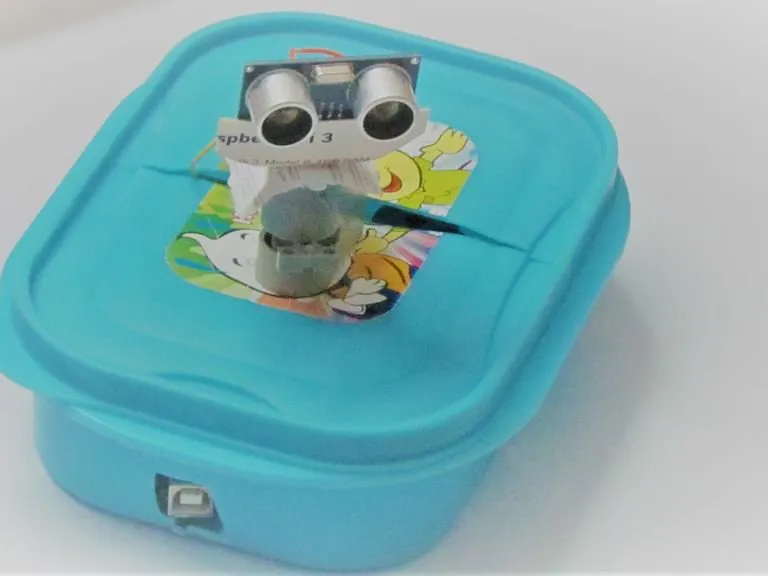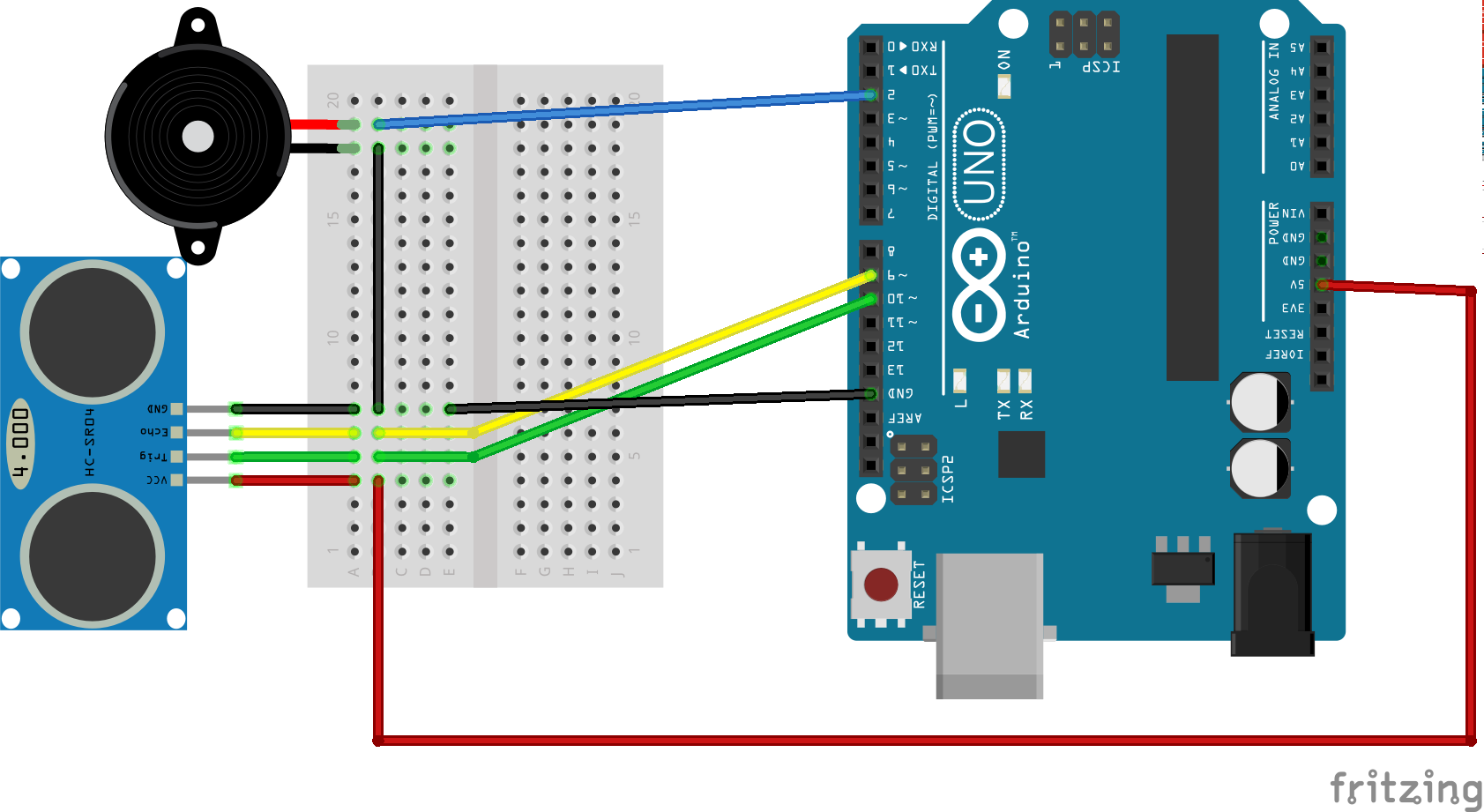Project tutorial# Arduino Based Security System © GPL3+

Do you want to build an easy security system? Well this is the perfect tutorial for you!

• 13,934 views
• 26 respects

 Knife

## Apps and online services

### Introduction

This project is a Arduino Based Security System consisting of a Arduino Uno, a servo motor, a ultrasonic sensor and a piezoelectric buzzer.

Here, the ultrasonic sensor senses an object that is within its range, which activates the buzzer. This sensor data is also fed into a Processing IDE software, which creates a graphical map.

The servo motor is used to rotate the ultrasonic sensor so as to cover a large area.

This is a pretty simple project and can be done within an hour.

### Here is the procedure for the project -

• Connect the Trigger Pin of the Sensor to Pin 2 of the Arduino
• Connect the Echo Pin of the Sensor to Pin 3 of the Arduino
• Connect Vcc and GND of the Sensor Supply and Ground respectively
• Place the buzzer on the breadboard
• Connect its Positive End to Pin 10 of the Arduino and connect the Negative End to ground
• Attach the black and red wire of the servo motor to ground and supply respectively
• Attach the yellow wire of the servo motor to Pin 9 of the Arduino
• Connect 5V terminal of the Arduino to supply and GND terminal of the Arduino to ground

After Finishing these connections, place the entire circuit inside the box as shown in the figure.

Then it's time to code.

## Code

##### Security System_IDEC9Search
```#include <Servo.h>.
int tPin = 2;
int ePin = 3;
int buz = 10;
int d1,d2;
int i =0;
int sdistance;
Servo myServo;
void setup() {
Serial.begin(9600);
pinMode(tPin, OUTPUT);
pinMode(ePin, INPUT);
pinMode(buz,OUTPUT);
myServo.attach(9);
}
void loop() {
for(i=0;i<=180;i += 10)
{
myServo.write(i);
delay(30);
d2 = calculateDistance();

Serial.print(i);
Serial.print(",");
Serial.print(d2);
Serial.print(".");
}

for(i=180;i>0; i-=10){
myServo.write(i);
delay(30);
d2 = calculateDistance();
Serial.print(i);
Serial.print(",");
Serial.print(d2);
Serial.print(".");
}
}
int calculateDistance(){

digitalWrite(tPin,HIGH);
delay(1000);
digitalWrite(tPin, LOW);
d1 = pulseIn(ePin, HIGH);
d2 = d1 * 0.0171821306;
if (d2 < 10)
{
digitalWrite(buz,HIGH);

}
else
{
digitalWrite(buz,LOW);
}
return d2;

}
```
##### ProcessingJava
```import processing.serial.*; // imports library for serial communication
import java.awt.event.KeyEvent; // imports library for reading the data from the serial port
import java.io.IOException;
Serial myPort; // defines Object Serial
// defubes variables
String angle="";
String distance="";
String data="";
String noObject;
float pixsDistance;
int iAngle, iDistance;
int index1=0;
int index2=0;
PFont orcFont;
void setup() {

size (1366, 768); // ***CHANGE THIS TO YOUR SCREEN RESOLUTION***
smooth();
myPort = new Serial(this,"COM3", 9600); // starts the serial communication
myPort.bufferUntil('.'); // reads the data from the serial port up to the character '.'. So actually it reads this: angle,distance.
}
void draw() {

fill(98,245,31);
// simulating motion blur and slow fade of the moving line
noStroke();
fill(0,4);
rect(0, 0, width, height-height*0.065);

fill(98,245,31); // green color
// calls the functions for drawing the radar
drawLine();
drawObject();
drawText();
}
void serialEvent (Serial myPort) { // starts reading data from the Serial Port
// reads the data from the Serial Port up to the character '.' and puts it into the String variable "data".
data = data.substring(0,data.length()-1);

index1 = data.indexOf(","); // find the character ',' and puts it into the variable "index1"
angle= data.substring(0, index1); // read the data from position "0" to position of the variable index1 or thats the value of the angle the Arduino Board sent into the Serial Port
distance= data.substring(index1+1, data.length()); // read the data from position "index1" to the end of the data pr thats the value of the distance

// converts the String variables into Integer
iAngle = int(angle);
iDistance = int(distance);
}
pushMatrix();
translate(width/2,height-height*0.074); // moves the starting coordinats to new location
noFill();
strokeWeight(2);
stroke(98,245,31);
// draws the arc lines
arc(0,0,(width-width*0.0625),(width-width*0.0625),PI,TWO_PI);
arc(0,0,(width-width*0.27),(width-width*0.27),PI,TWO_PI);
arc(0,0,(width-width*0.479),(width-width*0.479),PI,TWO_PI);
arc(0,0,(width-width*0.687),(width-width*0.687),PI,TWO_PI);
// draws the angle lines
line(-width/2,0,width/2,0);
popMatrix();
}
void drawObject() {
pushMatrix();
translate(width/2,height-height*0.074); // moves the starting coordinats to new location
strokeWeight(9);
stroke(255,10,10); // red color
pixsDistance = iDistance*((height-height*0.1666)*0.025); // covers the distance from the sensor from cm to pixels
// limiting the range to 40 cms
if(iDistance<10){
// draws the object according to the angle and the distance
}
popMatrix();
}
void drawLine() {
pushMatrix();
strokeWeight(9);
stroke(30,250,60);
translate(width/2,height-height*0.074); // moves the starting coordinats to new location
popMatrix();
}
void drawText() { // draws the texts on the screen

pushMatrix();
if(iDistance>10) {
noObject = "Out of Range";
}
else {
noObject = "In Range";
}
fill(0,0,0);
noStroke();
rect(0, height-height*0.0648, width, height);
fill(98,245,31);
textSize(25);

text("2cm",width-width*0.3854,height-height*0.0833);
text("4cm",width-width*0.281,height-height*0.0833);
text("6cm",width-width*0.177,height-height*0.0833);
text("10cm",width-width*0.0729,height-height*0.0833);
textSize(40);
text("Akshay6766 ", width-width*0.875, height-height*0.0277);
text("Angle: " + iAngle +" ", width-width*0.48, height-height*0.0277);
text("Distance: ", width-width*0.26, height-height*0.0277);
if(iDistance<40) {
text("        " + iDistance +" cm", width-width*0.225, height-height*0.0277);
}
textSize(25);
fill(98,245,60);
text("30",0,0);
resetMatrix();
text("60",0,0);
resetMatrix();
text("90",0,0);
resetMatrix();
text("120",0,0);
resetMatrix();
text("150",0,0);
popMatrix();
}
```

## Schematics#### Ultrasonic Security System

Project tutorial by Ivan

• 92,015 views
• 180 respects

#### Security System Using Arduino Bluetooth Camera

Project tutorial by amrmostaafaa

• 29,074 views
• 42 respects

#### RFID Based Automatic Door System

Project showcase by Robodia Technology

• 81,319 views
• 169 respects

#### Security System And Access Control With Arduino And RFID

Project tutorial by Sarath P

• 15,992 views
• 27 respects

#### Android App-Based Home Automation System Using IOT

Project tutorial by Team Autoshack

• 77,631 views